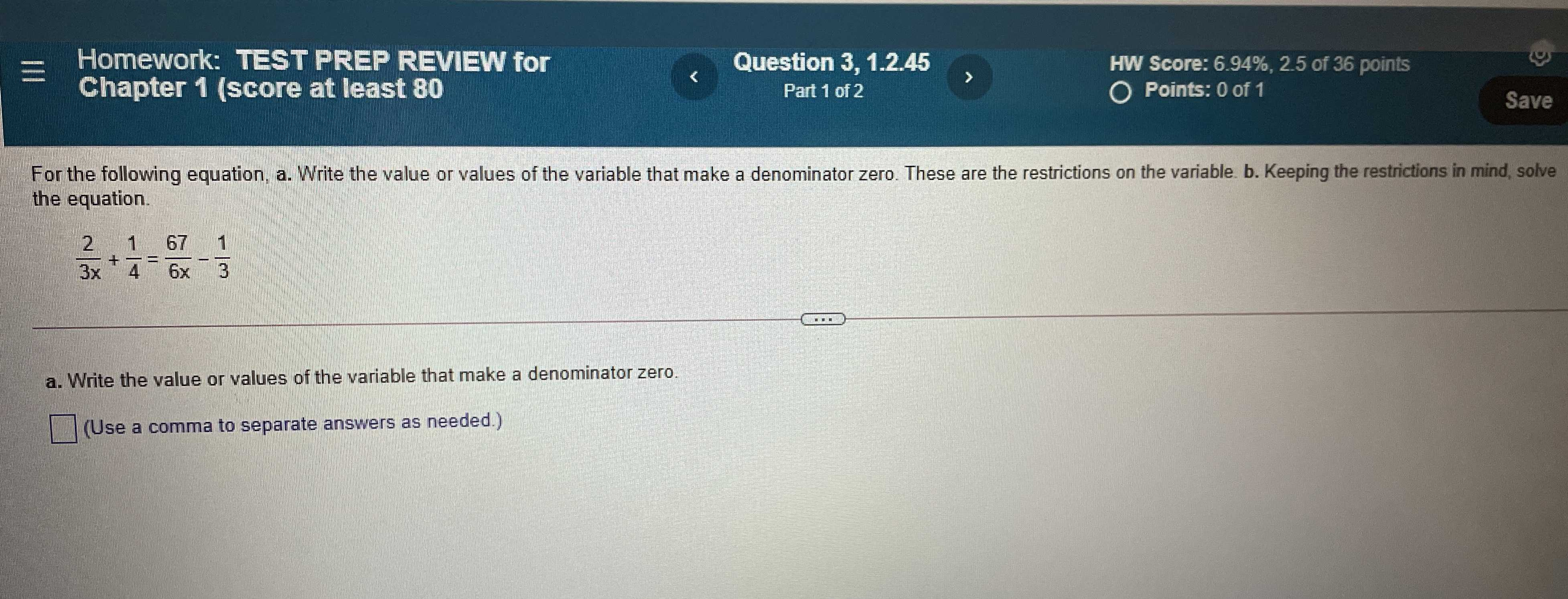### Still have math questions?

Algebra
QuestionFor the following equation, a. Write the value or values of the variable that make a denominator zero. These are the restrictions on the variable. b. Keeping the restrictions in mind, solve the equation.

$$\frac { 2 } { 3 x } + \frac { 1 } { 4 } = \frac { 67 } { 6 x } - \frac { 1 } { 3 }$$

a. Write the value or values of the variable that make a denominator zero.

$$\square$$ (Use a comma to separate answers as needed.)